# Methods and formulas for Fitted Line Plot

Select the method or formula of your choice.

## Polynomial regression models

### Formula

You can fit the following linear, quadratic, or cubic regression models:

Model type Order Statistical model
linear first Y = β0+ β1x + e
quadratic second Y = β0+ β1x + β2x2+ e
cubic third Y = β0+ β1x + β2x2+ β3x3+ e

Another way of modeling curvature is to generate additional models by using the log10 of x and/or y for linear, quadratic, and cubic models. In addition, taking the log10 of Y may be used to reduce right-skewness or nonconstant variance of residuals.

When Minitab fits the quadratic or cubic models, Minitab standardizes the predictors before it estimates the coefficients. The standardization reduces the multicollinearity among the predictors. The reduction ensures that the multicollinearity is so low that Minitab is unlikely to exclude any predictors from the model. The output shows the unstandardized coefficients in the original units of the predictors."

## Coefficient (Coef)

The formula for the coefficient or slope in simple linear regression is:

The formula for the intercept (b0) is:

In matrix terms, the formula that calculates the vector of coefficients in multiple regression is:

b = (X'X)-1X'y

### Notation

TermDescription
yiith observed response valuemean response
xiith predictor value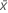mean predictor
Xdesign matrix
yresponse matrix

## S

### Notation

TermDescription
MSEmean square error

## R-sq

Another presentation of the formula is:

R2 can also be calculated as the squared correlation of y and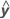.

### Notation

TermDescription
SSSum of Squares
yresponse variablefitted response variable

### Notation

TermDescription
MSMean Square
SSSum of Squares
DFDegrees of Freedom

## Degrees of freedom (DF)

The degrees of freedom for each component of the model are:

Sources of variation DF
Regression p
Error n – p – 1
Total n – 1

If your data meet certain criteria and the model includes at least one continuous predictor or more than one categorical predictor, then Minitab uses some degrees of freedom for the lack-of-fit test. The criteria are as follows:
• The data contain multiple observations with the same predictor values.
• The data contain the correct points to estimate additional terms that are not in the model.

### Notation

TermDescription
n number of observations
p number of coefficients in the model, not counting the constant

The sum of the squared distances. SS Regression is the portion of the variation explained by the model. SS Error is the portion not explained by the model and is attributed to error. SS Total is the total variation in the data.

SS Regression:
SS Error:
SS Total:

### Notation

TermDescription
yi i th observed response value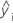i th fitted responsemean response

The Mean Square of the error (also abbreviated as MS Error or MSE, and denoted as s2) is the variance around the fitted regression line. The formula is:

### Notation

TermDescription
yiith observed response value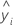ith fitted response
nnumber of observations
pnumber of coefficients in the model, not counting the constant

The formula for the Mean Square (MS) of the regression is:

### Notation

TermDescriptionmean responseith fitted response
pnumber of terms in the model

The formula for the total Mean Square (MS) is:

### Notation

TermDescriptionmean response
yiith observed response value
nnumber of observations

## F-value

The formulas for the F-statistics are as follows:

F(Regression)
F(Term)
F(Lack-of-fit)

### Notation

TermDescription
MS RegressionA measure of the variation in the response that the current model explains.
MS ErrorA measure of the variation that the model does not explain.
MS TermA measure of the amount of variation that a term explains after accounting for the other terms in the model.
MS Lack-of-fitA measure of variation in the response that could be modeled by adding more terms to the model.
MS Pure errorA measure of the variation in replicated response data.

## P-value – Analysis of variance table

The p-value is a probability that is calculated from an F-distribution with the degrees of freedom (DF) as follows:

Numerator DF
sum of the degrees of freedom for the term or the terms in the test
Denominator DF
degrees of freedom for error

1 − P(Ffj)

### Notation

TermDescription
P(Ff)cumulative distribution function for the F-distribution
ff-statistic for the test

## Residual (Resid)

### Notation

TermDescription
eii th residual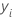i th observed response value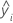i th fitted response
By using this site you agree to the use of cookies for analytics and personalized content.  Read our policy